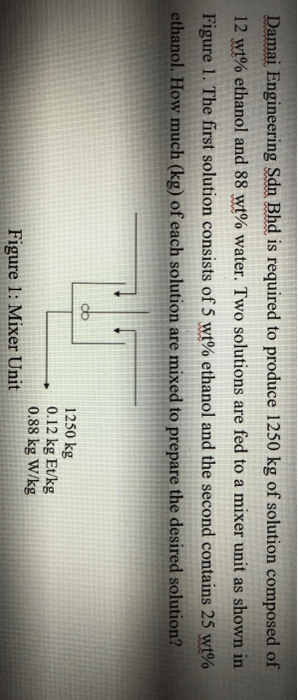# Damai Engineering Sdn Bhd is required to produce 1250 kg of solution composed of 12 wt% ethanol and 88 wt% water. Two solutions are fed to a mixer unit as shown in Figure 1. The first solution consists of 5 wt% ethanol and the second contains 25 wt% ethanol. How much (kg) of each solution are mixed to prepare the desired solution?

Question-AnswerCategory: Material And Energy BalanceDamai Engineering Sdn Bhd is required to produce 1250 kg of solution composed of 12 wt% ethanol and 88 wt% water. Two solutions are fed to a mixer unit as shown in Figure 1. The first solution consists of 5 wt% ethanol and the second contains 25 wt% ethanol. How much (kg) of each solution are mixed to prepare the desired solution?

Damai Engineering Sdn Bhd is required to produce 1250 kg of solution composed of 12 wt% ethanol and 88 wt% water. Two solutions are fed to a mixer unit as shown in Figure 1. The first solution consists of 5 wt% ethanol and the second contains 25 wt% ethanol. How much (kg) of each solution are mixed to prepare the desired solution?